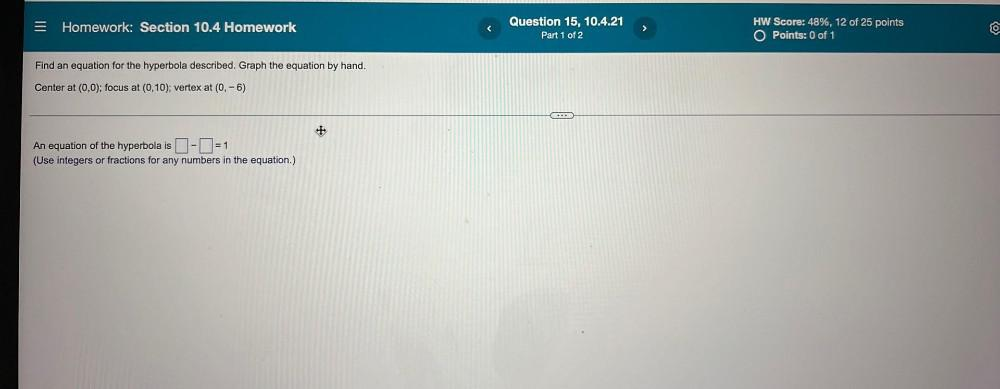Question:

# Homework: Section 10.4 Homework Question 15, 10.4.21 Part 1 of 2 > HW Score: 48%, 12 of 25 points O Points: 0 of 1 Find an equatHomework: Section 10.4 Homework Question 15, 10.4.21 Part 1 of 2 > HW Score: 48%, 12 of 25 points O Points: 0 of 1 Find an equation for the hyperbola described. Graph the equation by hand. Center at (0,0); focus at (0,10); vertex at (0, -6) ... + An equation of the hyperbola is -=1 (Use integers or fractions for any numbers in the equation.)